# WMF rare big vase Original Art Nouveau silver plated Albert Mayer metalware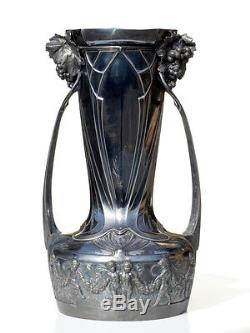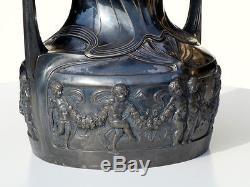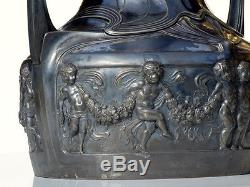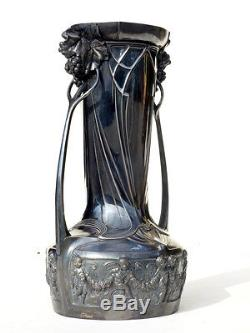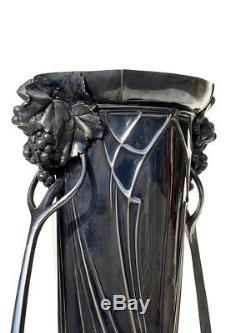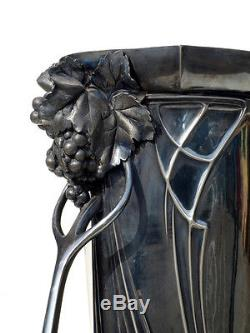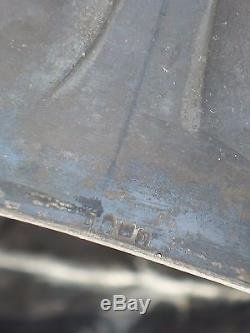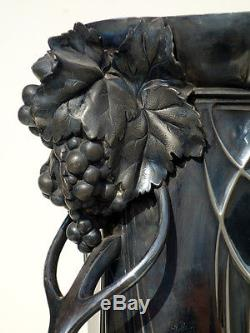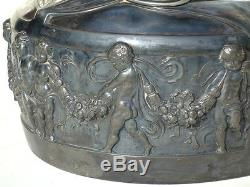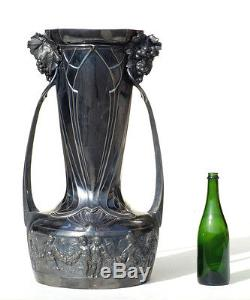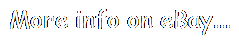A L B E R T M A Y E R. W M F 1 8 8 4 - 1 9 1 4. S t u n n i n g W M F v a s e. S i l v e r M e t a l.

V e r y G o o d C o n d i c t i o n. R a r e m o d e l. M E A S U R E S. H 7 0 x 3 7 c m. W E S H I P I N W O R L D W I D E.

I N G C O S T. I T A L Y. E U R O P E.

O T H E R. C O U N T R Y. P l e a s e. T E R M S O F S A L E. P A Y M E N T.

P a y p a l - B a n k T r a n s f e r. P R O F E S S I O N A L P A C K E T. B u b b l e W r a p - P o l y s t y r e n e c u b e s - H a r d C a r d b o a r d B o x e s. C O M M U N I C A T I O N. P r o m p t a n s w e r w i t h i n 2 4 h o u r s.

The item "WMF rare big vase Original Art Nouveau silver plated Albert Mayer metalware" is in sale since Monday, August 28, 2017. This item is in the category "Antiques\Periods & Styles\Art Nouveau". The seller is "tangram_xy07" and is located in Milano.

This item can be shipped worldwide.

• Maker: WMF
• Style: Art Nouveau
• Original/Reproduction: original early xx century
• Material: silver metal
• Designer: Albert Mayer
• Height: 70 cm